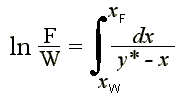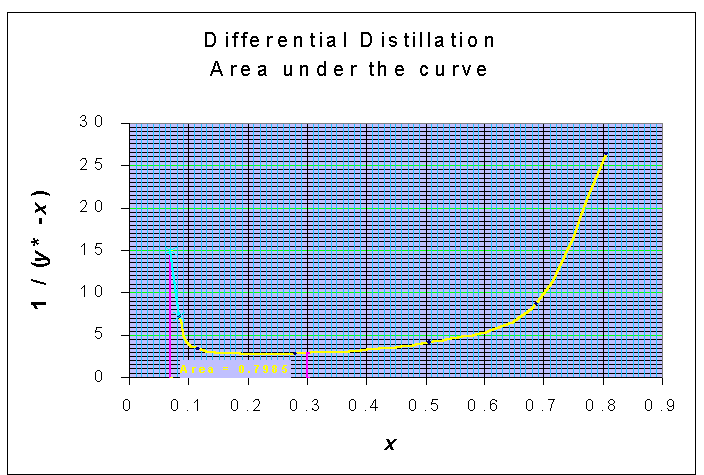### Differential Distillation

A liquid feed consisting of 1200 gmoles of mixture containing 30% naphthalene and 70% dipropylene glycol is differentially distilled at 100 mm Hg pressure and final distillate contains 55% of the feed solution. The VLE data are

 x 8.4 11.6 28 50.6 68.7 80.6 88 y 22.3 41.1 62.9 74.8 80.2 84.4 88
1. determine the amount of distillate
2. determine the concentration of naphthalene in residue and distillate

Calculations:

F = 1200 gmol

F = D + W

D = 0.55 x 1200 = 660 gmol

Amount of distillate, D = 660 gmol

W = 1200 - 660 = 540 gmol

From Rayleigh's equationxW is calculated from the graphical method, for which the integral term is the above equation is balanced:

ln (F/W) = ln (1200/540) = 0.7985The area of 0.7985 is obtained for xW = 0.07

F xF = D yD,avg + W xW

660 x  yD,avg = 1200 x 0.3 - 540 x 0.07

yD,avg = 0.4882

Concentration of naphthalene in residue = 7%

Concentration of naphthalene in distillate = 48.82%